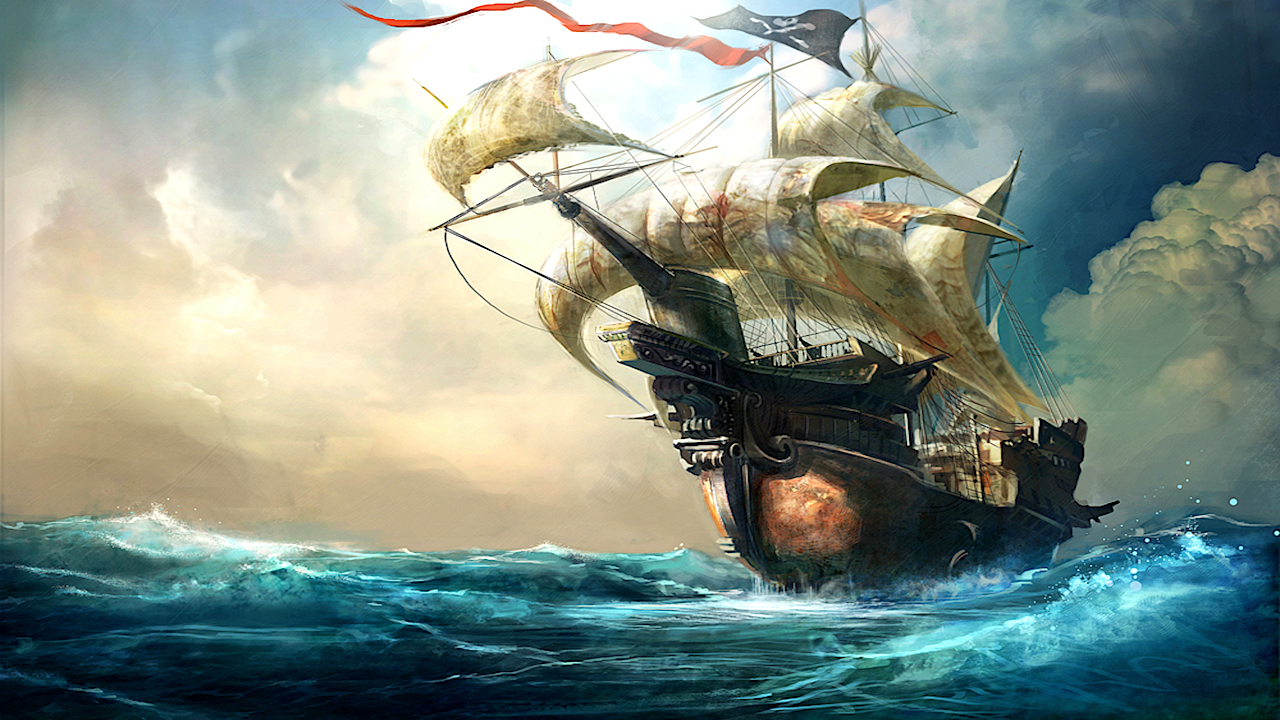# Naval BattleSchneider Pirates

##### Path of the Dragon
.The Schneider Pirates are a crew named after their captain. One of the other main members is a dog named Buzz with a devil fruit - the Tori Tori no Mi, Model: Eagle. This crew sails the seas for a good time, and they will not hesitate to attack anyone they deem a threat.

##### Path of the Dragon
.Schneider
Level 25

25 × 5 = 125
Human Bonus: 4+4+4+6 in Str, Spd, Vit, M.A.
Strength: 37+4=41
Agility: 37+4=41
41×2 = 82
-Reaction: 41
-Movement: 41
Vitality: 17+4=21
21×2=42
-Endurance: 21
-Resilience: 21
Fighting Style: 19+6=25
25×2=50
-Brawling: 50Buzz
Level 30

30 × 5 = 150
Human Bonus: 6+6+6+9 in Str, Spd, Vit, M.A.
Strength: 35+6=41
Agility: 35+6=41
41×2=82
-Reaction: 41
-Movement: 41
Vitality: 35+6=41
41×2=82
-Endurance: 41
-Resilience: 41
Tori Tori no Mi, Model: Eagle: 45+9=54Ante
Level 20

20 × 5 = 100
Human Bonus: 4+4+4+6 in Str, Spd, Vit, M.A.
Strength: 27+4=31
Agility: 17+4=21
21×2=42
-Reaction: 21
-Movement: 21
Vitality: 17+4=21
21×2=42
-Endurance: 21
-Resilience: 21
Fighting Style: 24+6=31
31×2=62
-Sword: 31
-Brawling: 31

Schneider Pirates(15)
Level 15

15 x 5 = 75
Strength: 20+2=22
Agility: 15+2=17
17x2=34
-Reaction:17
-Movement:17
Vitality:20+2=22
22x2=4
-Resilience:22
-Endurance:22
Fighting Style: 20
20x2=40
-Swordmanship: 40